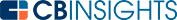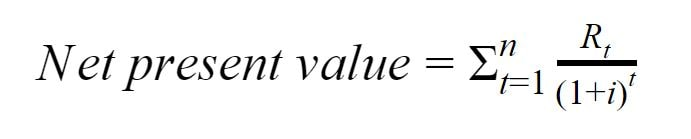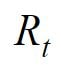# What is the NPV Formula in Excel?

Net present value (NPV) is the current value of all the cash flows that will happen in the future. This is calculated with the nominal value of cash flow in the future and a discount rate. The equation can be used to calculate the net present value of all cash flows in the future.

## Why is NPV useful?

According to the time value of money, money in the future is worth less than money right now. Due to inflation and other macroeconomic conditions, the buying power of the same amount of money decreases over time. This is evident from the increasing prices for goods of the same exact brand increasing year over year. By calculating NPV, you can work out whether a project or investment will produce a net profit or a loss.

## How to calculate NPV

The assumption for using the equation is that the cash flow during a period happens only once. One year is the period generally used in calculations. The equation for calculating net present value is:Here,       is the net cash flow for period t. Net negative cash flow for a period is represented as a negative number and a net positive cash flow is represented as a negative number. i is the discount rate used for the calculation. Use the appropriate discount rate in calculations. If the time period is taken as a year, use the yearly discount rate. If the time period is a month, use the monthly discount rate. n is the total number of periods.## NPV in Excel

From the equation for NPV, you can see that when the number of periods becomes large, the calculation becomes cumbersome. Even with a scientific calculator, it’s difficult to calculate the NPV when a large number of periods are involved. Excel offers a simple function to make this calculation easier. The syntax for using the NPV function in Excel is:

= NPV (rate, value1, value2, ...)

Here, rate is the discount rate for the period. value1 is the cash flow for the first period, value2 is the cash flow for the second period, and so on until the last period. All the values for a period have to be given as inputs.

Any periods without cash flow should be entered as 0. For example, if the second period has no cash flow, do not use the net cash flow of the third period for value2. You should input value2 as 0, otherwise there will be an error in the final result. Here’s an example in Excel:Notice that even though the cash flow in the third term is 0, it is not skipped. It is marked as 0 so there are no errors in the final result.In this example, the net present value of the cash flows for the seven years at a discount rate of 8% is \$35,318.

You may also like# www.cbinsights.com